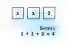# Python Sum Example

Use the sum method on a list of integers. Understand when TypeError is caused by sum.

Sum. A list contains the numbers 10, 20 and 5: its sum is 35. We can a for-loop to iterate and add up the element values. Or we can use a simpler built-in method.Two arguments. The sum() built-in receives 2 arguments. The first is an iterable collection like list—these elements are summed. The second argument is added to the sum.

An example. Here we introduce a list with 3 integers in it. We sum those integers with the sum() built-in method. And then we use sum with 2 arguments.
Argument 1: An iterable like a list we want to sum. The list must have summable elements like integers, not sub lists.
Argument 2: A value that is added to the sum of the iterable's elements. We can use this argument to sum previous sums.Python program that uses sum, 2 arguments values = [1, 1, 2] # The sum of the 3 values is this number. result = sum(values) print(result) # Use sum with 2 arguments. # ... The second argument is added to the element sum. result = sum(values, 1000) print(result) Output 4 1004

Error in summing. We cannot sum nested iterables. Instead sum() only counts up top-level elements in our list. A nested list leads to a TypeError.List, strings
Python program that uses sum, nested list error # This list has a nested list element. # ... It cannot be summed with sum. values = [10, [20, 30]] # This will cause a TypeError. result = sum(values) print(result) Output Traceback (most recent call last): File "C:\programs\file.py", line 9, in <module> result = sum(values) TypeError: unsupported operand type(s) for +: 'int' and 'list'

Fsum example. The sum() built-in will work with float numbers like 1.5. But it may return a less-accurate result than the math.fsum specialty method.Math: fsum

A review. Why use sum? Why not just loop over elements and add them up? In some programs, sum() leads to simpler, more maintainable code, and this causes fewer bugs.

Home

© 2007-2020 sam allen. send bug reports to info@dotnetperls.com.